Courses

# Test: Electrostatic Potential Energy

## 10 Questions MCQ Test Physics Class 12 | Test: Electrostatic Potential Energy

Description
This mock test of Test: Electrostatic Potential Energy for JEE helps you for every JEE entrance exam. This contains 10 Multiple Choice Questions for JEE Test: Electrostatic Potential Energy (mcq) to study with solutions a complete question bank. The solved questions answers in this Test: Electrostatic Potential Energy quiz give you a good mix of easy questions and tough questions. JEE students definitely take this Test: Electrostatic Potential Energy exercise for a better result in the exam. You can find other Test: Electrostatic Potential Energy extra questions, long questions & short questions for JEE on EduRev as well by searching above.
QUESTION: 1

### A particle of mass 1 kg and charge 1/3μC is projected towards a non conducting fixed spherical shell having the same charge uniformly distributed on its surface. The minimum initial velocity V0 of projection of particle required if the particle just grazes the shell is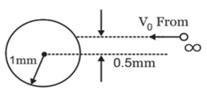Solution:

From conservation of angular momentum,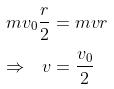from conservation of energy,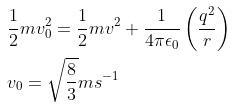QUESTION: 2

Solution:

Definition based

QUESTION: 3

### Negative mutual potential energy corresponds to attraction between two charges

Solution:

Definition based

QUESTION: 4

The amount of work done in moving a charge from one point to another along an equipotential line or surface charge is

Solution:

Since Potential difference between two points in equipotential surfaces is zero, the work done between two points in equipotential surface is also zero.

QUESTION: 5

In bringing an electron towards another electron, the electrostatic potential energy of the system

Solution:

Definition based

QUESTION: 6

The potential energy of a system containing only one point charge is

Solution:
QUESTION: 7

A proton is accelerated from rest through a potential of 500 volts. Its final kinetic energy is

Solution:

K = qV

QUESTION: 8

The electrostatic potential energy between two charges q1 and q2 separated by a distance by r is given by

Solution:

We know that energy required/gained = force x displacement
As F = kqQ / r2 and displacement is r
We get U = kQq /r
Where k = 1/4ϵ

QUESTION: 9

What is the electrostatic potential energy of a system containing two charges 40 nC and 60 nC at a distance of 36 cm?

Solution:

Definition based

*Answer can only contain numeric values
QUESTION: 10

Three point charges Q, +q and +q are placed at the vertices of a right-angled isosceles triangle as shown in the figure. If the net electrostaic energy of the configuration is zero, find the value Q\q is [Take √2 = 1.4]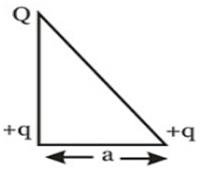Solution:

Net electrostatic energy of the configuration will be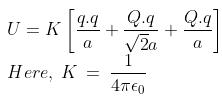Putting U = 0 we get,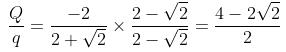-(2-√2) = -0.6